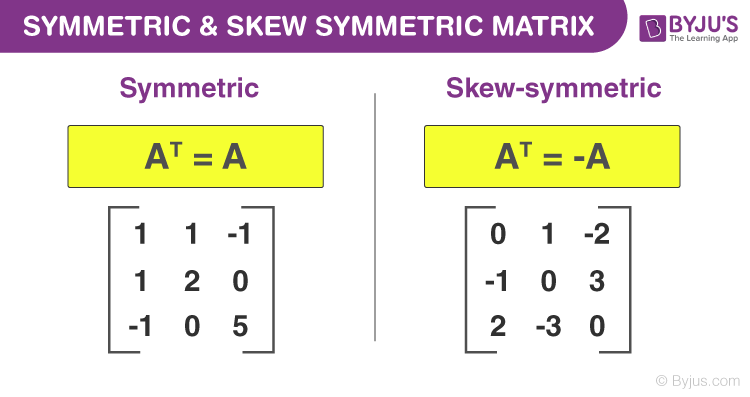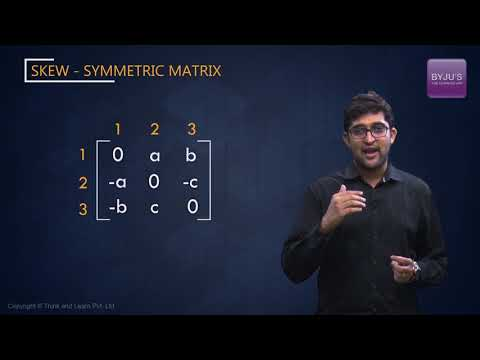# Symmetric Matrix & Skew Symmetric Matrix

A symmetric matrix and skew-symmetric matrix both are square matrices. But the difference between them is, the symmetric matrix is equal to its transpose whereas skew-symmetric matrix is a matrix whose transpose is equal to its negative.

If A is a symmetric matrix, then A = AT  and if A is a skew-symmetric matrix then AT = – A.

## Symmetric Matrix

To understand if a matrix is a symmetric matrix, it is very important to know about transpose of a matrix and how to find it. If we interchange rows and columns of an m×n  matrix to get an n × m   matrix, the new matrix is called the transpose of the given matrix. There are two possibilities for the number of rows (m) and columns (n) of a given matrix:

• If m = n, the matrix is square
• If m ≠ n, the matrix is rectangularFor the second case, the transpose of a matrix can never be equal to it. This is because, for equality, the order of the matrices should be the same. Hence, the only case where the transpose of a matrix can be equal to it, is when the matrix is square. But this is only the first condition. Even if the matrix is square, its transpose may or may not be equal to it. For example:

$$\begin{array}{l}If~ A =\begin{bmatrix}1& 2\cr3 & 4\end{bmatrix}, then~ A’ =\begin{bmatrix}1& 3\cr2 & 4\end{bmatrix}.\end{array}$$

Here, we can see that A ≠ A’.

Let us take another example.

$$\begin{array}{l}B =\begin{bmatrix}1& 2&17\cr2 & 5&-11 \cr17&-11&9\end{bmatrix} \end{array}$$

If we take the transpose of this matrix, we will get:

$$\begin{array}{l} B’ =\begin{bmatrix}1& 2&17\cr2 & 5&-11 \cr17&-11&9\end{bmatrix} \end{array}$$

We see that B = B’. Whenever this happens for any matrix, that is whenever transpose of a matrix is equal to it, the matrix is known as a symmetric matrix. But how can we find whether a matrix is symmetric or not without finding its transpose? We know that:

$$\begin{array}{l}If~ A = [a_{ij}]_{m\times n},\ then~ A’ = [a_{ij}]_{n\times m} \text{( for all the values of i and j )}\end{array}$$

So, if for a matrix A, aij = aji (for all the values of i and j) and m = n, then its transpose is equal to itself. A symmetric matrix will hence always be square. Some examples of symmetric matrices are:

$$\begin{array}{l} P = \begin{bmatrix} 15& 1\cr 1 & -3 \end{bmatrix} \end{array}$$

$$\begin{array}{l} Q = \begin{bmatrix} -101 & 12 & 57\cr 12 & 1001 & 23 \cr 57 & 23 & -10001 \end{bmatrix} \end{array}$$

## Properties of Symmetric Matrix

• Addition and difference of two symmetric matrices results in symmetric matrix.
• If A and B are two symmetric matrices and they follow the commutative property, i.e. AB =BA, then the product of A and B is symmetric.
• If matrix A is symmetric then An is also symmetric, where n is an integer.
• If A is a symmetrix matrix then A-1 is also symmetric.

## Skew Symmetric Matrix

A matrix can be skew symmetric only if it is square. If the transpose of a matrix is equal to the negative of itself, the matrix is said to be skew symmetric. This means that for a matrix  to be skew symmetric,

A’=-A

Also, for the matrix, aji =-aij(for all the values of i and j). The diagonal elements of a skew symmetric matrix are equal to zero. This can be proved in following way:

The diagonal elements are characterized by the general formula,

aij , where i = j

If i = j, then aij = aii = ajj

If A is skew symmetric, then

aji = – aji

⇒ aii = – aii

⇒ 2.aii = 0

⇒ aii = 0

So, aij = 0 , when i = j  (for all the values of i and j)

Some examples of skew symmetric matrices are:

$$\begin{array}{l} P = \begin{bmatrix} 0 & -5\cr 5 & 0 \end{bmatrix} \end{array}$$

$$\begin{array}{l} Q = \begin{bmatrix} 0 & 2&-7\cr -2 & 0&3 \cr 7 & -3 &0 \end{bmatrix} \end{array}$$

### Properties of Skew Symmetric Matrix

• When we add two skew-symmetric matrices then the resultant matrix is also skew-symmetric.
• Scalar product of skew-symmetric matrix is also a skew-symmetric matrix.
• The diagonal of skew symmetric matrix consists of zero elements and therefore the sum of elements in the main diagonals is equal to zero.
• When identity matrix is added to skew symmetric matrix then the resultant matrix is invertible.
• The determinant of skew symmetric matrix is non-negative

### Determinant of Skew Symmetric Matrix

If A is a skew-symmetric matrix, which is also a square matrix, then the determinant of A should satisfy the below condition:

Det (AT) = det (-A) = (-1)n det(A)

The inverse of skew-symmetric matrix does not exist because the determinant of it having odd order is zero and hence it is singular.

### Eigenvalue of Skew Symmetric Matrix

If A is a real skew-symmetric matrix then its eigenvalue will be equal to zero. Alternatively, we can say, non-zero eigenvalues of A are non-real.

Every square matrix can be expressed in the form of sum of a symmetric and a skew symmetric matrix, uniquely. Learn various concepts in maths & science by visiting our site BYJU’S.

## Video LessonQ1

### What is a symmetric matrix?

A symmetric matrix is a square matrix that is equal to transpose of itself. If A is a symmetric matrix, then it satisfies the condition: A = AT
Q2

### How do you know if a matrix is symmetric?

To know if a matrix is symmetric, find the transpose of that matrix. If the transpose of that matrix is equal to itself, it is a symmetric matrix.

Q3

### Give an Example of a Matrix Which is Symmetric but not Invertible.

A zero (square) matrix is one such matrix which is clearly symmetric but not invertible.

Q4

### Is Symmetric Matrix Diagonalizable?

Yes, a symmetric matrix is always diagonalizable.

Q5

### What is skew-symmetric matrix?

A skew-symmetric matrix is a square matrix whose transpose equals to its negative. It should satisfy the below condition:
AT = – A
If aij represents the value of i-th row and j-th column, then the skew symmetric matrix condition is given by;
aij = -aji
Q6

### What is the difference between symmetric and skew-symmetric matrix?

The transpose of the symmetric matrix is equal to the original matrix.
The transpose of skew symmetric matrix is equal to negative of the original matrix.
Test your knowledge on What Is Symmetric Matrix And Skew Symmetric Matrix